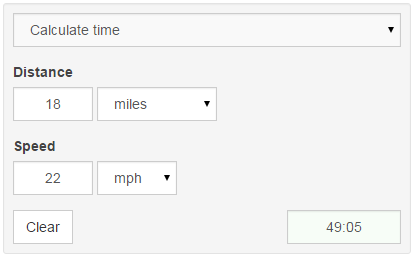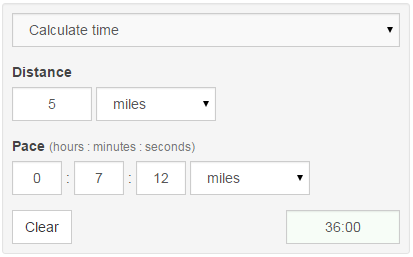If you have a support crew of family and friends coming to watch you race, you’re likely to be faced with questions like these:

“How long will it take you to finish?” “When can we see you get off the bike?”

Seeing your family and friends during a race can be a huge boost mentally. You don’t want to miss seeing them, or worse, have them miss you finish. So, how do you predict your times? If you are training with a device you should have a good idea of your average bike speed and running pace. Knowing those numbers along with race distances will allow you to predict your finish times.

To predict time, we need to know two variables, distance and rate. With these variables, we can use a mathematical formula to calculate time. Time equals distance divided by rate (t = d/r). Here’s where the pace and speed calculators come into play. They’ll do all the calculations for you, all you need to do is enter the distance and average rate and it’ll give you the time it’ll take to complete.

You’ll want to use the pace calculator to predict your run time since running rate is typically measured by how much time it takes to complete a distance, e.g. 8:12 per mile. Use the speed calculator to predict your cycling time since cycling rate is measured by miles per hour (mph) or kilometers per hour (km/h).

#### Predicting Bike Times

Let’s take a look at an example. We’ll start with “When can we see you get off the bike?” Say the race I’m doing has an 18 mile bike leg and I think I can average 22mph for that distance. Since we are talking about cycling time, we should use the speed calculator. Make sure the speed calculator is set to “Calculate time”. Enter the distance for the bike portion of your race (18 miles). Then enter your average speed (22mph). Voila, the calculator gives you the time it’ll take you to complete the bike portion of the race (49:05).#### Predicting Run Times

Predicting run times is just as easy. Let’s figure out how long it will take me to complete the 5 mile run portion of my race. I’ll say that I can average 7:12 per mile. Since we are calculating run time, we need to use the pace calculator. Make sure it is set to “Calculate time”. Enter the distance (5 miles). Then, enter the pace (7:12). It tells me that I’ll complete the run portion in 36 minutes.#### Predicting Swim Times

All we need to do now in order to answer the “When will you finish?” question is to calculate the amount of time it will take us to do the swim. Swimming is a little trickier to calculate because I only give you kilometers and miles for distance units and swim rate is typically calculated in meters and in some cases yards. If you know you can swim 100 meters in 56 seconds, you’ll have to convert meters to kilometers in order to use the pace calculator. Let’s use that pace to figure out how long it would take me to complete a 800 meter swim. For this, I’ll use the pace calculator. Make sure it’s set to “Calculate time”. For distance, I need to convert 800 meters to kilometers, so I’ll divide 800 by 1000, which gives me 0.8. For pace, I need to convert the time it takes me to do 100 meters to the time it takes me to do 1 kilometer, so I’ll take 56 * 10 = 560 seconds. Since the input boxes for time aren’t that restricting, I can enter 560 seconds for my pace and choose kilometers. After entering my info, it tells me that I will complete the 800 meter swim in 7:28.#### Putting It All Together

Now that we have all of our times, we just need to estimate our transition times and add them all together to get our overall race time prediction.

7:28 (swim) + 01:18 (T1) + 49:05 (bike) + 00:56 (T2) + 36:00 (run) = 1 hour, 34 minutes, and 47 seconds.